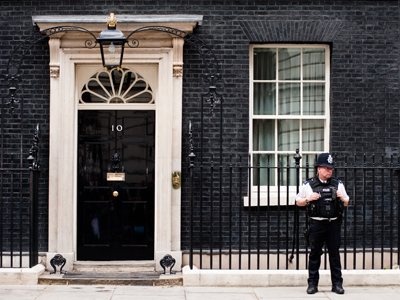The Prime Minister lives at 10 Downing Street, but what is a prime number?

# Pick a Figure 3

This is the third in our series of Eleven Plus Verbal Reasoning quizzes on Picking a Figure to Fulfil Criteria. By now you should be a dab hand at finding multiples, factors, prime numbers and squares. Make sure you understand these terms as you’ll need to know what they mean when you come to take your exam.

Square numbers are the result of multiplying a number by itself. You probably know most of the lower ones already but, just in case you need a reminder:

1 x 1 = 1, 2 x 2 = 4, 3 x 3 = 9, 4 x 4 = 16, 5 x 5 = 25, 6 x 6 = 36, 7 x 7 = 49, 8 x 8 = 64, 9 x 9 = 81, 10 x 10 = 100, 11 x 11 = 121, 12 x 12 = 144

If you can memorise these it will be a great help, in your maths lessons, in your 11+ exams, and in your future life. Many people think that maths is no use to them, but you’d be surprised just how often we make use of it. Now on with the quiz. Good luck!

Example:
Which of the following is not a factor of 24?

2, 6, 4, 48, 12 Answer:
‘Factor’ means a number which fits exactly into another. The numbers 2, 4, 6 and 12 all fit into 24 whereas 48 does not, so the correct answer is 48.
1.
Which of the following is not a multiple of 13?

52, 39, 26, 91, 83
83
52
91
39
Multiples are numbers you find after multiplying another number. More simply, if a number is in another number’s times table, then it is a multiple of that number. To quickly see if a number is a multiple of another, divide it by the other number and, if there is no remainder, it is a multiple.
All of the options, except 83, can be divided by 13 with no remainder
2.
Which of the following is a factor of 77?

21, 14, 11, 28, 9
21
14
11
9
Factors are numbers which divide into another number without leaving a remainder. The factors of 77 are 1, 7, 11 and 77. The only one of these which appears amongst the options is 11
3.
Which of the following is not a factor of 38?

76, 19, 2, 1, 38
38
76
19
2
Factors are numbers which divide into another number without leaving a remainder. The factors of 38 are 1, 2, 19 and 38. The number 76 is not a factor of 38, but 38 is a factor of 76
4.
Which of the following is a prime number?

77, 11, 9, 15, 4
4
77
9
11
Prime numbers can only be divided by two numbers (one and themselves) without leaving a remainder. They will only appear in the 1 times table and their own times table, but in no others. The number 11 is the only option which can meet these requirements
5.
Which of the following is not a multiple of 5?

20, 105, 553, 25, 90
553
105
20
90
Multiples are numbers you find after multiplying another number. More simply, if a number is in another number’s times table, then it is a multiple of that number. To quickly see if a number is a multiple of another, divide it by the other number and, if there is no remainder, it is a multiple.
All of the options, except 553, can be divided by 5 with no remainder (any number ending with a 0 or a 5 is a multiple of 5)
6.
Which of the following is not a prime number?

31, 27, 17, 47, 5
31
17
5
27
Prime numbers can only be divided by two numbers (one and themselves) without leaving a remainder. They will only appear in the 1 times table and their own times table, but in no others. All of the options, except 27 (which appears in the 3 and 9 times tables) meet these requirements
7.
Which of the following is a multiple of 11?

132, 122, 155, 67, 34
122
155
132
67
Multiples are numbers you find after multiplying another number. More simply, if a number is in another number’s times table, then it is a multiple of that number. To quickly see if a number is a multiple of another, divide it by the other number and, if there is no remainder, it is a multiple.
The only one of the options which can be divided by 11 with no remainder is 132
8.
Which of the following is not a square number?

225, 400, 100, 200, 144
100
200
144
400
Square numbers are what we get when we multiply a number by itself. All of the options are square numbers (10 x 10 = 100, 12 x 12 = 144, 15 x 15 = 225, 20 x 20 = 400) but 200 is not
9.
Which of the following is a factor of 89?

3, 89, 31, 21, 17
17
3
21
89
Factors are numbers which divide into another number without leaving a remainder. The factors of 89 are 1 and 89 (which means it is a prime number). The only one of these amongst the options is 89 – every number is a factor and a multiple of itself
10.
Which of the following is a square number?

66, 147, 169, 97, 110
66
169
110
97
Square numbers are what we get when we multiply a number by itself. 169 is the square of 13 because 13 x 13 = 169. None of the other options are square numbers
Author:  Stephen O'Hara and Ian Miles